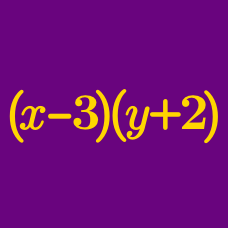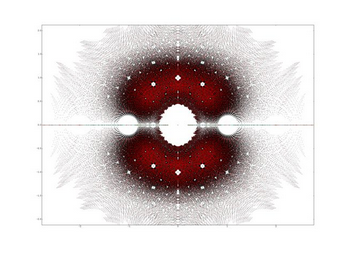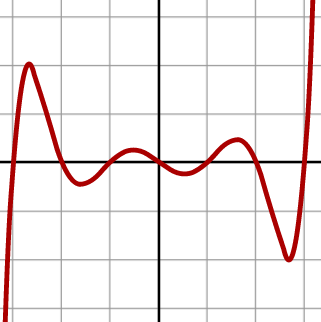Algebra

# Polynomial Arithmetic: Level 4 Challenges

Let $P(x) : (x - 1)(x - 2)(x - 3) \dots\ (x - 50)$

Let $Q(x) : (x + 1)(x + 2)(x + 3) \dots\ (x + 50)$

If $P(x)Q(x) = a_{100}x^{100} + a_{99}x^{99} + \ldots\ + a_{1}x^1 + a_0,$ then compute $a_{100} -a_{99} - a_{98} - a_{97}.$Suppose the polynomials $P_1(x)$ and $P_2(x)$ are obtained by expanding and simplifying the following algebraic expressions

$P_1(1) = (1 + x^2 - x^5)^{2000} \\ P_2(x) = (1 - x^2 + x^5)^{2000}.$

Let $C_1$ and $C_2$ be the coefficient of $x^{800}$ in the polynomials $P_1(x)$ and $P_2(x)$ respectively. Which of the given option is true?

Let $T(x)=x^{124}+x^{123}+x^{122}+\ldots+x+1$ and $a_{1},a_{2},a_{3}, \ldots, a_{123},a_{124}$ be the values of $x$ for which $T(x) = 0$.

If $V_{n} = a_{1}^{n} + a_{2}^{n} + a_{3}^{n} + \ldots + a_{124}^{n}$, then find

$\large \sum_{n=0}^{100000} (-1)^{n}V_{n}$What is the minimum value of $p(2)$ if the following 4 conditions are followed?

1. $p(x)$ is a polynomial of degree $17$.

2. All roots of $p(x)$ are real.

3. All coefficients are positive.

4. The coefficient of $x^{17}$ is 1.

5. The product of roots of $p(x)$ is -1.

##### Image Credit: Wikimedia Septic Graph

$P(x)$ is a polynomial with integral coefficients such that the absolute value of the constant term of $P(x)$ is smaller than 1000. Given further that $P(19)=P(94)=1994$, find the constant term of the polynomial $P(x)$.

×